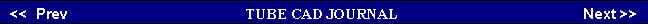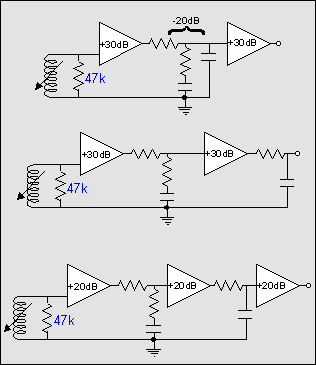Tube Phono PreampsSeveral topologies & tricksPart 2 of 2Part one of this article covered active RIAA equalization. Active means the frequency response is tailored to fit that of the RIAA curve   by varying the amount of the feedback signal returned to the input (i.e. based on frequency). The problems arising from the use of feedback were covered--potential instability and the need for extra gain to power the feedback mechanism.   Passive Equalization      The alternative to active equalization is passive equalization. Passive RIAA equalization means brute force equalization: the frequency response is tailored to fit that of the RIAA curve by varying the amount of attenuation at different frequencies through a complex RC network. The advantages: no voltage overload, no feedback, no instability problems, and no high frequency gain limits. The disadvantages: no gain (in fact there is usually some slight insertion loss beyond the required frequency tailoring) and possible impedance matching problems.     We will examine the many forms that passive equalization can take: two gain stages with one complete equalization network in between, two gain stages with one partial-equalization network in between and one partial equalization network following the last gain stage, or three gain stages with one partial-equalization network in between each gain stage.
 Gain-Equalization-Gain     In the topmost circuit we see the approach of using two gain stages with one complete RIAA equalization network in between. One 6922 or 12AX7 or 6N1P per channel is all that is needed. If grounded-cathode amplifiers are used, a plate resistor and a bypassed cathode resistor, are all that is missing. Refinements might include eliminating the cathode resistors altogether by using batteries to bias the triodes. Further refinements might entail using a truly high-voltage power supply, say a 1000-volt B+ with a staggered turn-on; or maybe using a truly low high-voltage power supply, say 100 volts with solid-state constant current source loading of the triodes. Somewhat paradoxically, both of these approaches yield the same results: high gain and good power supply noise rejection.     Alternatively, the generic grounded-cathode amplifier topology can be retained, but the number of triodes in parallel can be increased to 2 or 4 or even 6 or 8. The following schematic illustrates this approach. The circuit is as straightforward as can be imagined, the only twist being the many triodes (four in the first stage and two in the last) used in parallel.